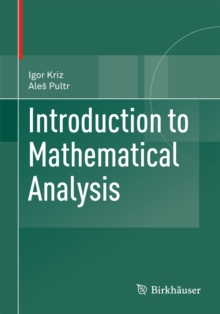Supporting your high street Find out how »
• My AccountIntroduction to Mathematical Analysis Paperback / softback

Description

The book begins at the level of an undergraduate student assuming only basic knowledge of calculus in one variable.

It rigorously treats topics such as multivariable differential calculus, Lebesgue integral, vector calculus and differential equations.

After having built on a solid foundation of topology and linear algebra, the text later expands into more advanced topics such as complex analysis, differential forms, calculus of variations, differential geometry and even functional analysis.

Overall, this text provides a unique and well-rounded introduction to the highly developed and multi-faceted subject of mathematical analysis, as understood by a mathematician today.

Information

• Format: Paperback / softback
• Pages: 510 pages, 1 Illustrations, color; XX, 510 p. 1 illus. in color.
• Publisher: Springer Basel
• Publication Date:
• Category: Algebra
• ISBN: 9783034806350

Other Formats

£64.99

£53.75

on all orders

Pick up orders

from local bookshops

£64.99

£55.24